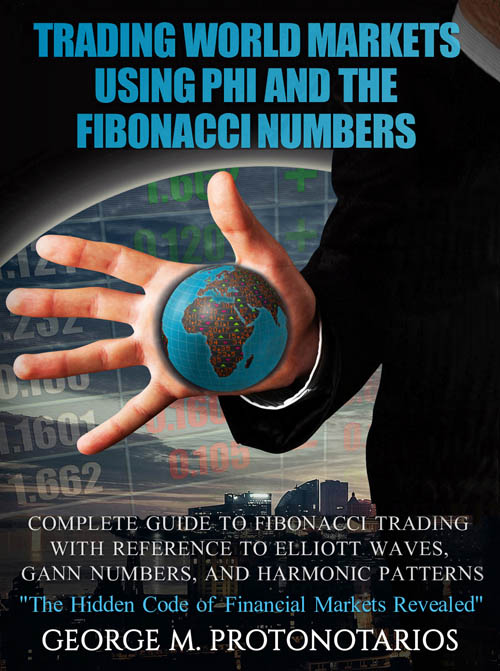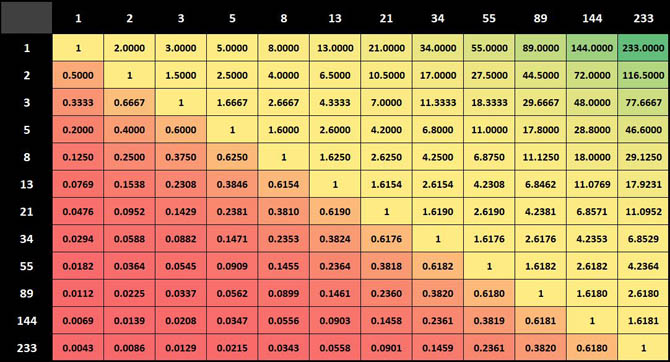TRADING WORLD MARKETS USING PHI AND THE FIBONACCI NUMBERSSubtitle: Complete Guide to Fibonacci Trading With Reference to Elliott Waves, Gann Numbers, and Harmonic Patterns

AuthorGeorge M. Protonotarios

Description:

The complete guide to Fibonacci trading and Phi with reference to Elliott Waves, Dow Theory, Gann Numbers, and Harmonic Patterns, for trading successfully the Global Financial Markets (Forex currencies, Stocks, Indices, Metals, and Energies).

This Book covers an enormous range of trading theories and methodologies involving the Fibonacci numbers and their products. You will find all the basic Fibonacci trading practices and tools based on Fibonacci ratios in it. Phi and the Fibonacci numbers do not form just another tool of technical analysis. Phi proportions are everywhere: in arts, architecture, our DNA’s helix spiral, and even in our nature’s plant formations.

The first chapter begins with the mathematical properties of Phi and several of its applications outside the financial markets. In the next few chapters, you will find information about the Dow Theory, the Elliott Wave Theory, and the Gann numbers. At the end of each chapter, you will be able to detect the correlation of each theory with the Fibonacci numbers. You will learn also how you to use the Fibonacci numbers in order to create a trading system based on Fibonacci Moving Averages (MAs). In addition, you will find information about some popular Fibonacci trading tools such as the Fibonacci Retracement, the Fibonacci Extension, and the Fibonacci Fan. There are two chapters dedicated to Harmonic Trading and Harmonic Patterns. Harmonic trading is one of the most sophisticated trading practices and it is entirely based on Fibonacci proportions. Six basic harmonic patterns are presented with their properties and charts with examples. The last chapter is dedicated to money management and the effect of the irrational brain in our everyday decision-making process.

By combining the information and tools presented in all chapters you have the chance to build the foundations of a trading system out of chaos. A trading system that can make you less emotional when trading the global markets and significantly improve your odds of winning.INTRODUCTION

CHAPTERS:

1. THE ORIGINS OF FIBONACCI NUMBERS AND PHI (Φ)
2. THE DOW THEORY AND FIBONACCI NUMBERS
3. THE ELLIOTT WAVE THEORY AND FIBONACCI NUMBERS
4. W. D. GANN AND FIBONACCI NUMBERS
5. FIBONACCI NUMBERS AND MOVING AVERAGES
6. FIBONACCI RETRACEMENT AND OTHER TOOLS
8. SIX HARMONIC PATTERNS
9. THE IRRATIONAL BRAIN & MONEY MANAGEMENT

APPENDIX

1. Dow Jones Industrial Record Highs
2. Heikin-Ashi Charts

■ Trading World Markets Using Phi and the Fibonacci Numbers

Qexpert.com